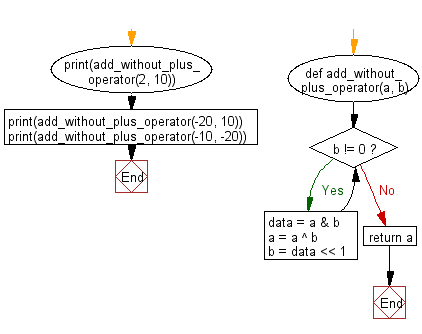﻿ Python: Add two positive integers without using the '+' operator - w3resource# Python: Add two positive integers without using the '+' operator

## Python Basic - 1: Exercise-14 with Solution

Write a Python program to add two positive integers without using the '+' operator.

Note: Use bitwise operations to add two numbers.

Sample Solution:

Python Code:

``````def add_without_plus_operator(a, b):
while b != 0:
data = a & b
a = a ^ b
b = data << 1
return a
``````

Sample Output:

```12
-10
-30
```

Pictorial Presentation:Flowchart:## Visualize Python code execution:

The following tool visualize what the computer is doing step-by-step as it executes the said program:

Python Code Editor :

Have another way to solve this solution? Contribute your code (and comments) through Disqus.

What is the difficulty level of this exercise?

Test your Python skills with w3resource's quiz

﻿

## Python: Tips of the Day

Python: Debug With the Print() Function

```>>> for i in range(5):
...     print(i, end=', ' if i < 4 else '\n')
...
0, 1, 2, 3, 4
>>> for i in range(5):
...     print(f'{i} & {i*i}', end=', ' if i < 4 else '\n')
...
0 & 0, 1 & 1, 2 & 4, 3 & 9, 4 & 16
```